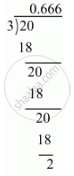Share

# Express the Following Rational Number as Decimal: 2/3 - CBSE Class 9 - Mathematics

ConceptIntroduction of Real Number

#### Question

Express the following rational number as decimal:

2/3

#### Solution

Given rational number is 2/3

Now we have to express this rational number into decimal form. So we will use long division methodTherefore 2/3=0.6666

rArr2/3=0.bar6

Is there an error in this question or solution?

#### APPEARS IN

Solution Express the Following Rational Number as Decimal: 2/3 Concept: Introduction of Real Number.
S